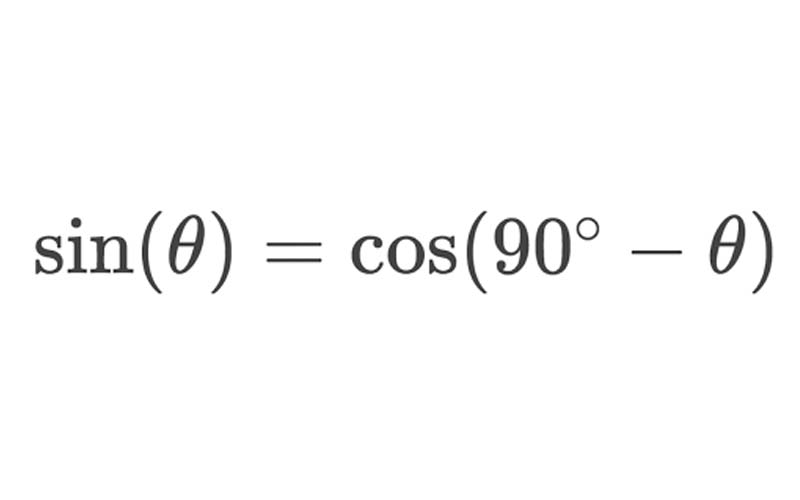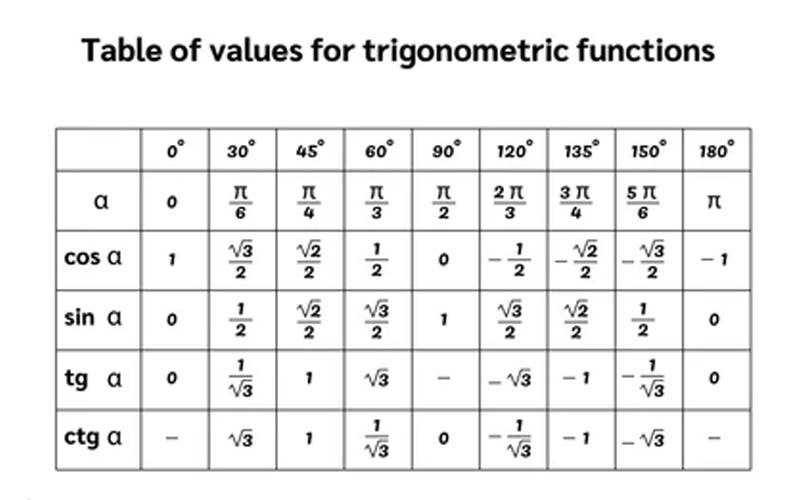# How do you Convert Sin to Cos? Where are they used?Trigonometry is a branch of mathematics that deals with the relationship between the sides and angles of a triangle.

The word Trigonometry is derived from the Greek word trigonon and metron. Which means triangle and measure respectively.

The trigonometric ratios are widely used for finding the angles and missing sides of triangles.

## Important Trigonometric Functions

The trigonometric ratios of a triangle are also called trigonometric functions.

The three important trigonometric functions are sine, cosine, and tangent. They are also known as sine cos and tan.

## Trigonometric FunctionsLet us consider a right-angled triangle where the longest side is called the hypotenuse, the vertical side is called opposite or perpendicular and the horizontal side is called Adjacent or base.

## Six Trigonometric Function

There are six trigonometric functions: Sine, Cosine, Tangent, Cosecant, Secant, and Cotangent.

## Formula to calculate

Sine (Sin) : Opposite/Hypotenuse ( p/h) Cosine (Cos): Opposite/ Adjacent ( p/b) Tangent (Tan): Adjacent/ Hypotenuse ( b/h) Cosecant (Cosec): Hypotenuse/Opposite ( h/p) Secant (Sec): Hypotenuse/ Adjacent (h/b) Cotangent (Cot): Adjacent/Opposite ( b/p) How to convert Sin to Cos?

Sin (degree) = Cos ( 90° – degree)

Cos (degree) = Sin (90° – degree)

## Daily use of Trigonometry

Have you ever studied Trigonometry and then wondered why we have to study this? what is the use of trigonometry in daily life?

Well, let us help you to understand this trigonometry in daily life.

Trigonometry is not directly used in our daily life. But there are various things that we love that are based on trigonometric functions.

Let’s find out more?

## Measuring heights of Mountains and Buildings

Trigonometric functions can be used to measure the height of buildings or mountains.

If you know the distance between where you are observed, and the angle of elevation you can easily find the height of the building.

## Video games

Do you love playing video games? Moreover, like the classic Mario. Well, Mario jumps over obstacles using trigonometric functions.

The gaming industry uses various trigonometric functions to develop games. i

## Sound Industry

The Sound Industry also used various Trigonometry functions to measure sound waves and frequency.

## Construction

Trigonometry is widely used in Construction. It is used while making parallel walls, perpendiculars, roof inclinations, or measuring the Height of Buildings, etc.

## Flight engineering

Flight engineering uses trigonometry to measure speed, distance direction along with the speed and direction of the wind.

## Physics

trigonometry is used to find components of vectors, waves, both physical and electromagnetic oscillations, etc.

Even in projectile motion, there’s a lot of application of trigonometry.

## Police

Police also use a trigonometric calculation to measure how a car accident happened or to know the angle of bullets shot, or to know how the object falls.

## Marine biologists

They also use trigonometry to measure how light levels are different in different lands which affects the ability of algae to photosynthesize.

They also use trigonometry to find the distance between celestial bodies, etc.

#### Conclusion

Trigonometry is an unbreakable part of Mathematics. It is used by different fields. Gaming is a very important one of them.

I hope you got a better understanding of trigonometry with this article.